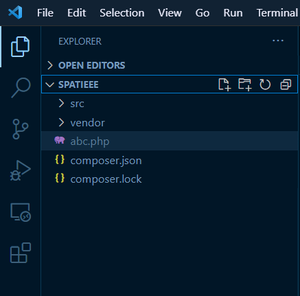GeeksforGeeks App
Open AppBrowser
Continue

# How to make async functions in PHP ?

Let us understand what is the meaning of Async (Asynchronous):

A program does not need to be executed line by line. The program calls the function and moves further (does not wait for the function to return). It will execute in the background. Non-Blocking methods are executed asynchronously. Async allows the parallel execution of the code. Async is also used to make faster execution of code and allow us the execution of multiple task.

Async Function in PHP:

• In synchronous programming, if we want to read some file and return its content other functions block until the current function returns.
• But an asynchronous programming program calls the function and moves further and all the same is happening in the background.
• To write the Async program in PHP, we need to libraries (ReactPHP and Amp) which is used to implement non-blocking I/O in php.
• AMP is a concurrency framework for php and allows us to manage event loops, async iterators, and promises.

Before going to the code, let’s first understand the functions provided by AMP:

1. then() Function: The then() function is executed only when the block of code in Async is successfully executed and we can perform operations on the result returned by Async.

Syntax:

```then(function () {
// You can write operations here..
})```

2. timeout() Function: The timeout() function occurs when the php request takes longer time than usual and it does not need a parameter.

Syntax:

```timeout(function () {
// Execute when request takes longer time to execute.
})```

3. catch Block: Exceptions are handled in the catch. When the program throws an exception(error) catch block will be executed.

Syntax:

```catch(function (\$exception) {
// Here we can handle exceptions
})```

4. await() Function: The await function allows us to wait until async returns.

Syntax:

`await(\$promise);`

5. Promise: A promise represents a single result of an asynchronous operation.

`\$promise = \$deferred->promise();`

The steps required for “Async Code” are given below:

Step 1: You have to install composer to import libraries(ReactPHP and Amp).

Step 2: Initialize the composer using the following command.

`composer init`

Step 3: Create .php file. Your Project Structure should look like this.Step 4: To implement the Async function we need to import the package first by using composer.

`composer require amphp/react-adapter`

Step 5: Import class present inside AMP.

## PHP

 ``

Let us take a few examples to understand this concept better:

Example 1: In asynchronous programming, the program calls the function and moves further and allows the execution of multiple tasks. For eg: If we first write the table of 2 and then we write some print functions, then the print function will execute first due to non-blocking I/O.

## PHP

 ````` ` ```    ````    ````    `````` ` ```    ``

``        ``GeeksforGeeks``    ``

`` ` `    ``addtimer(1, ``function``(){``        ``foreach` `(range(1, 5) ``as` `\$i``) {``            ``\$output` `= ``\$i` `* 2;``            ``echo` `" "``, ``\$output` `. ``"
"``. ``" "``;``        ``}``        ``echo` `" After Table"``. ``"
"``. ``" "``;``    ``});`` ` `    ``echo` `" After Loop Time"``. ``"
"``. ``" "``;``    ``\$loop``->run();``    ``?>```` ` ``

Output:Example 2: Reading text file using Async. We can read a file in a non-blocking asynchronous way, where the program calls the function and move to another function, does not wait for the function to return. When the file is completely read then it is printed.

## PHP

 ````` ` ```    ````    ````    `````` ` ```    ``

``        ``GeeksforGeeks``    ``

`` ` `    ``addtimer(1, ``function``(){``        ``\$file` `= ``fopen``(``"amar.txt"``, ``"r"``);``            ``while``(!``feof``(``\$file``)) {``                ``echo` `fgets``(``\$file``). ``"
"``. ``" "``;``            ``}``        ``echo` `" After Reading File "``. ``"
"``. ``" "``;``    ``});`` ` `    ``echo` `" After Loop Time"``. ``"
"``. ``" "``;``    ``\$loop``->run();``    ``?>```` ` ``

Output:My Personal Notes arrow_drop_up
Related Tutorials